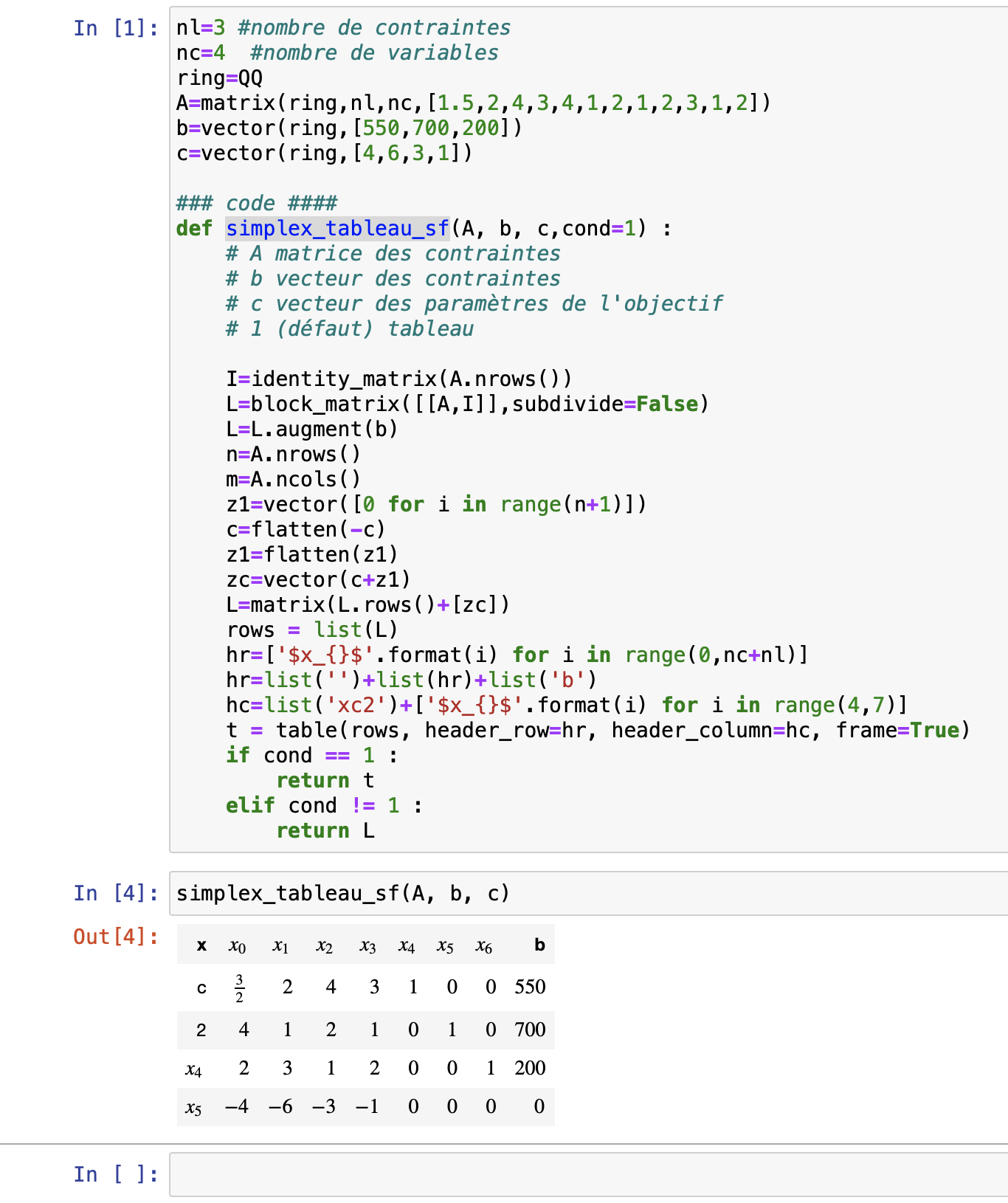# Unbrackable blanck

In the following code I am obliged to insert / to inside my fonction to obtain the desired result.

nl=3 #nombre de contraintes
nc=4  #nombre de variables
ring=QQ
A=matrix(ring,nl,nc,[1.5,2,4,3,4,1,2,1,2,3,1,2])
b=vector(ring,[550,700,200])
c=vector(ring,[4,6,3,1])

### code ####
def simplex_tableau_sf(A, b, c,cond=1) :
# A matrice des contraintes
# b vecteur des contraintes
# c vecteur des paramètres de l'objectif
# 1 (défaut) tableau

I=identity_matrix(A.nrows())
L=block_matrix([[A,I]],subdivide=False)
L=L.augment(b)
n=A.nrows()
m=A.ncols()
z1=vector([0 for i in range(n+1)])
c=flatten(-c)
z1=flatten(z1)
zc=vector(c+z1)
L=matrix(L.rows()+[zc])
rows = list(L)
hr=['$x_{}$'.format(i) for i in range(0,nc+nl)]
hr=list('')+list(hr)+list('b')
hc=list('xc2')+['$x_{}$'.format(i) for i in range(4,7)]
if cond == 1 :
return t
elif cond != 1 :
return L


So is there a blank which can be used as a string

edit retag close merge delete

Can you clarify? It is not clear what you are asking. In Python, ' ' is a blank string. In LaTeX, '~' is a blank.

John look the difference when I use hr=list('/')+list(hr)+list('b'), hr=list(' ')+list(hr)+list('b') and hr=list('/')+list(hr)+list('b'). The only case where I obtain a correct result is the first and I want to have a blanc space not a \.

What happens if you use ' ' rather than ''?

Sorry John, I send the wrong code. I have changed it in the question. I hope now it is correct. My problem is only with the /. Now with ' ', it works. I don't know why earlier, I have a wrong result.

Quick comment: you can replace elif cond != 1 : with else:

Sort by » oldest newest most votedHere is a screenshot of what your code produces for me (using Sage 9.2):If I change '' to '/', it adds an extra column to the header row, so that the column "b" has nothing beneath it. Is that really what you want?

more